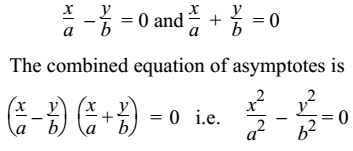# Asymptotes

An asymptote to a curve is the tangent to the curve such that the point of contact is at infinity. the asymptote touches the curve at +∞ and −∞.

Equations of Asymptotes to Hyperbola

There are two asymptotes to the hyperbola whose equations are

y = ±bx/a